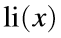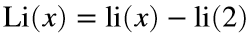# Problem 46066. Evaluate the logarithmic integral

The logarithmic integralplays a role in number theory because the related functionprovides an estimate for the number of primes less than x. MATLAB's Symbolic Toolbox has the function logint, but it is not available in basic MATLAB or Cody.
Write a function to evaluate the logarithmic integral.

### Solution Stats

21.21% Correct | 78.79% Incorrect
Last Solution submitted on Apr 29, 2023

### Community Treasure Hunt

Find the treasures in MATLAB Central and discover how the community can help you!

Start Hunting!